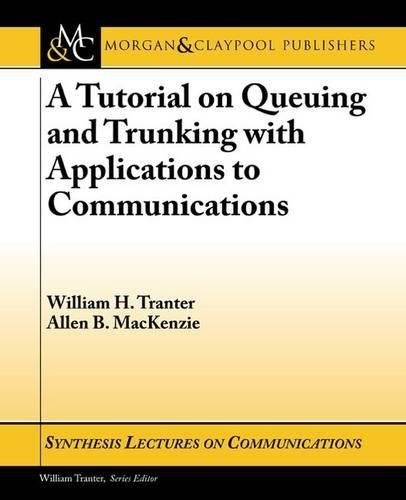•# A Tutorial on Queuing and Trunking with Applications to Communications - Synthesis Lectures on Communications (Paperback)

(author), (author)
£40.50
Paperback 104 Pages / Published: 01/11/2012
• We can order this

Usually dispatched within 2 weeks

The motivation for developing this synthesis lecture was to provide a tutorial on queuing and trunking, with extensions to networks of queues, suitable for supplementing courses in communications, stochastic processes, and networking. An essential component of this lecture is MATLAB-based demonstrations and exercises, which can be easily modified to enable the student to observe and evaluate the impact of changing parameters, arrival and departure statistics, queuing disciplines, the number of servers, and other important aspects of the underlying system model. Much of the work in this lecture is based on Poisson statistics, since Poisson models are useful due to the fact that Poisson models are analytically tractable and provide a useful approximation for many applications. We recognize that the validity of Poisson statistics is questionable for a number of networking applications and therefore we briefly discuss self-similar models and the Hurst parameter, long-term dependent models, the Pareto distribution, and other related topics. Appropriate references are given for continued study on these topics.

The initial chapters of this book consider individual queues in isolation. The systems studied consist of an arrival process, a single queue with a particular queuing discipline, and one or more servers. While this allows us to study the basic concepts of queuing and trunking, modern data networks consist of many queues that interact in complex ways. While many of these interactions defy analysis, the final chapter introduces a model of a network of queues in which, after being served in one queue, customers may join another queue. The key result for this model is known as Jackson's Theorem. Finally, we state the BCMP Theorem, which can be viewed as a further extension of Jackson's Theorem and present Kleinrock's formula, which can be viewed as the network version of Little's Theorem.

Publisher: Morgan & Claypool Publishers
ISBN: 9781598292688
Number of pages: 104
Weight: 198 g
Dimensions: 235 x 187 x 5 mm